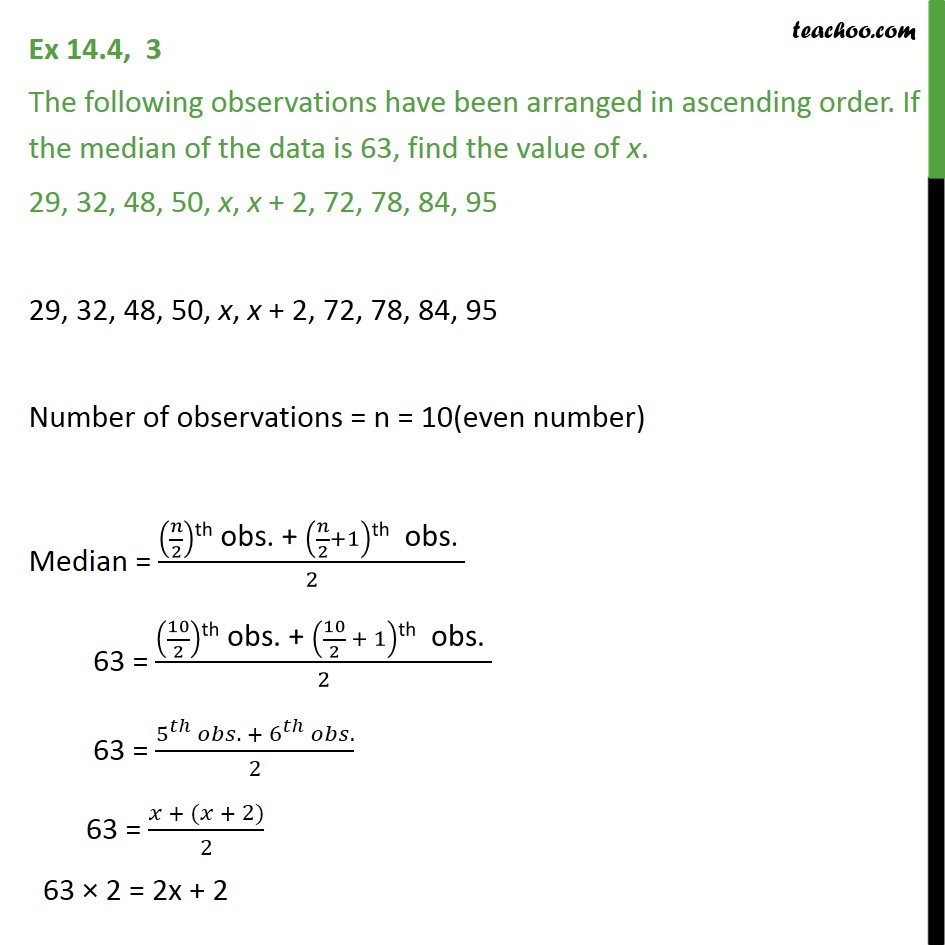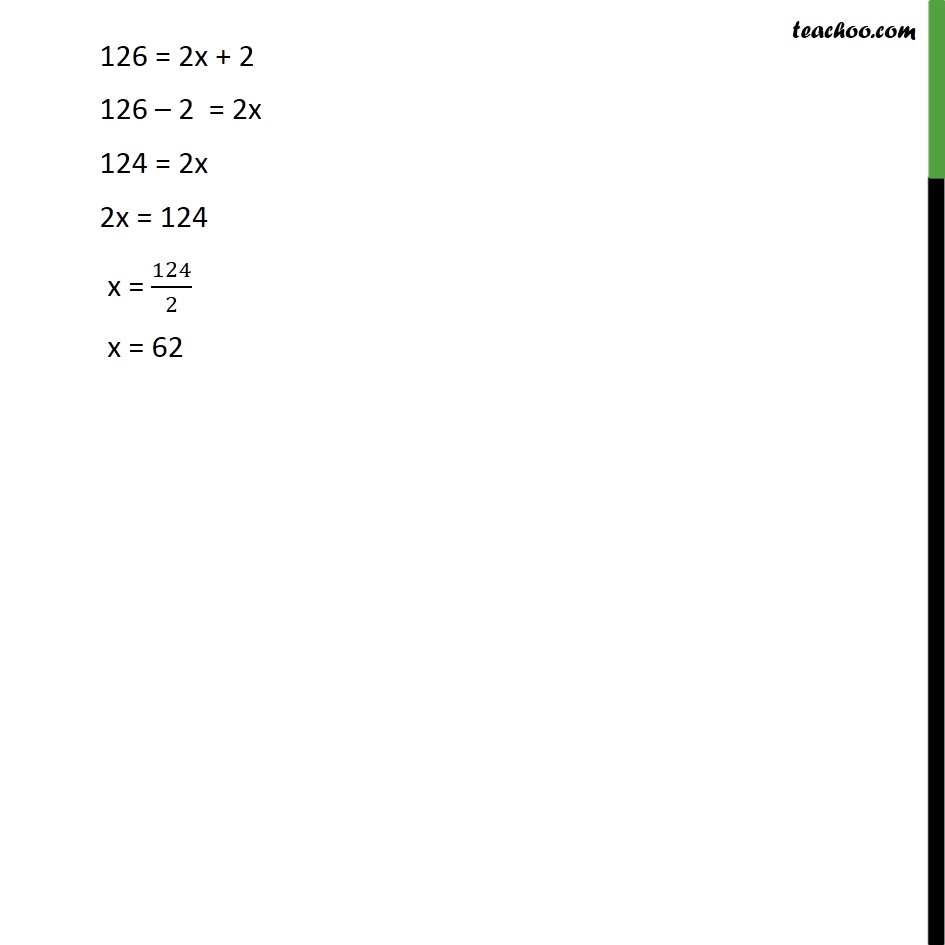Finding Mean, Median, Mode

Chapter 12 Class 9 Statistics
Serial order wiseLearn in your speed, with individual attention - Teachoo Maths 1-on-1 Class

### Transcript

Question 3 The following observations have been arranged in ascending order. If the median of the data is 63, find the value of x. 29, 32, 48, 50, x, x + 2, 72, 78, 84, 95 29, 32, 48, 50, x, x + 2, 72, 78, 84, 95 Number of observations = n = 10(even number) Median = ( /2)"th obs. + " ( /2+1)"th obs. " /2 63 = (10/2)"th obs. + " (10/2 + 1)"th obs. " /2 63 = (5^ . + 6^ .)/2 63 = ( + ( + 2))/2 63 2 = 2x + 2 126 = 2x + 2 126 2 = 2x 124 = 2x 2x = 124 x = 124/2 x = 62